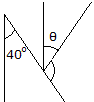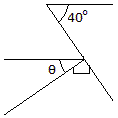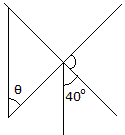# Engineering Mechanics - General Principles - Discussion

### Discussion :: General Principles - General Questions (Q.No.3)

3.Determine the angle:

 [A].= 30° [B].= 40° [C].= 60° [D].= 50°

Explanation:

No answer description available for this question.

 Anish Kur said: (Dec 1, 2010) In fig 2 ,considering the alternate angles we get 40+x+90=180 x=50,where x=theta(required angle)

 Sd Afzal said: (Dec 17, 2010) How please explain?

 Prasad said: (Jan 8, 2011) The first figure, I guess is wrong. The other angle other than 40 degree and theta is supposed to be perpendicular. It is moreover confusing.

 Karthik said: (Jan 14, 2011) Could'nt understand.

 Naga Chaitanya said: (Feb 9, 2011) Anish kur's explanation to second diagram is correct.

 Yuvaraj said: (Feb 10, 2011) Look at the 3rd fig. Extend that 40degree line to opposite direction, then the right portion of that 4 0degree line can be written as, 40+x+40=180 so x=100 which is vertically opposite to one of the angle in the Triangle, so the total angle in the triangle is θ+100+y=180, So θ+y=180 But why is vertically opposite to the angle 40 hence θ=40 ?

 Manpreet said: (Feb 19, 2011) The answer is in the third diagram..when the perpendicular line of 40 angle extends towards upper side then it clearly shows that angles should be 40 & 50..so thita will be 50 because of angle is made at same line.

 Jatinder Singh Tumber said: (Apr 20, 2011) Now look at first figure the line of 40 and theta are parallel and the common line of 40 and theta i.e. from right side of theta to right side of 40 is angle of 90, so left side of theta is anle of 40 so 90 - 40 =50.

 Neeraja said: (Apr 30, 2011) In the second fig since alternate angles are equal 40=90-theta then theta=90-40 =50

 M.Prakash said: (Jul 4, 2011) How please explain?

 Kiran said: (Jul 15, 2011) IN FIG 1 40+90+ THE ANGLE THAT IS 40+90+ANGLE =180 THERE FOR ANGLE = 50 THAT'S IT........ SIMPLE

 Thirupathaiah said: (Jul 24, 2011) 40 degrees is given vertically according to alternative angle beside the theta angle is 40 degrees we know that t+40=90 then t=50 degrees where t is theta

 Brahmananda Reddy said: (Jul 25, 2011) In third fig we have an ang bisector. So we get 40 again. Ang bisector divides 80 into 40 each. So from alternate angles principle we will get ans as 40.

 Damodaran said: (Aug 13, 2011) Still I'm not clear could you explain me in more in detail.

 Sathish Kumar said: (Aug 30, 2011) From the fig (90-x)+40=180 x=180-(90+40) Hence x=50

 Rajasekhar said: (Oct 23, 2011) Sum of triangle = 180 from fig 1 the angle specified next to theta is 40 opposite exterior angles are equal which is 40 x+90+40=180 x=180-130=50

 Rajanishree Ks said: (Nov 11, 2011) Kindly mention the details of the error here... see nohin error here act its right ans. watch fig 3, 90'=50'+40' thats it..:)

 Akanksha said: (Nov 30, 2011) In fig 1 40+90+x=180 x=50 In fig 2 , alternate angles we get 40+x+90=180 x=50,

 Darpan said: (Dec 1, 2011) In geometry there is one rule in that case the angle given other than thita is equal to 40. So for theta 90-40=50.

 Udayan Singh said: (Dec 27, 2011) In fig1- if unmentiond angle is90 then theta =5: fig2: theta+90+40=ans. Fig3: the angle besides the 40 must b a rit angle then theta =501 net ans for theta in thrice case to b 50.

 Manish Yadav said: (Feb 9, 2012) In first figure, angle b/w two line is 90 then 40+90+x=180. X=50.

 Desingu said: (Feb 28, 2012) In third figure, if you just remove the 40 degree line (only imagination)you can see right angle triangle, so that 90-40=50 degree. As per the rules is 50 degree

 Karthik said: (Apr 5, 2012) IN fig1 consider triangle ABC the angle A was 40 B was 90 then C WAS 50 now we draw perpendicular line to B 90 - 50=40 incliantion line angle 40 so 90 -40=50=theata.

 Er. Jagdeep Yadav said: (Sep 24, 2012) In 2nd fig. One angle is 90degree. One angle is alternate which is 40 degree. So total is 180 on a line then. 90+40+x=180. X=180-130. X=50.

 Himanth Venati said: (Jan 28, 2014) From fig 2: 90+40+x = 180. 130+x = 180. x = 50.

 Lakshmi said: (May 10, 2014) In 1st figure: The two lines are parallel hence it is 40. Since it is a straight line it makes 180. Hence 180-40 = 140. Theta = 140-90 = 50. Same in the second and third figure.

 Edy said: (Aug 15, 2014) Total Angle 1 Triangle = 180. Know One Angle 40. So 40 + 90 + X = 180. X = 180 - 90 + 40. X = 180 - 130. X = 50.

 N.Sakthi said: (Sep 10, 2015) I cannot understand.

 Nkayawa Muhidini said: (Nov 26, 2015) Okay I understanding that question but I need more explain.

 Manali Chowdhury said: (May 31, 2017) In the triangle one is 90° angle, and the same another angle of 40° in the same direction is also a 40° angle because it is placed on the same line. So, the other angle is θ. So, as we know the hole angle of a triangle is 180°. Therefore, solution, 40° + θ +90° = 180°. Or, θ + 90° = 180° - 40°. Or, θ + 90° = 140°. Or, θ= 140° - 90°. Or, θ = 50°. So, the angle θ is 50°. Thanking you.